Home » Wiki » Variable Frequency Drive for Constant Torque Loads

# Variable Frequency Drive for Constant Torque Loads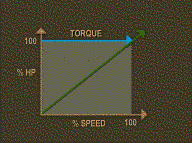Constant torque loads require the same amount of torque at low speeds as at high speeds. Torque remains constant throughout the speed range, and the horsepower increases and decreases in direct proportion to the speed.

Constant torque loads include most positive displacement and reciprocating pumps and compressors as well as traction drives and conveyors.

With constant torque loads, the torque is not a function of speed. As speed is changed, the load torque will remain fairly constant and the horsepower will change linearly with the speed.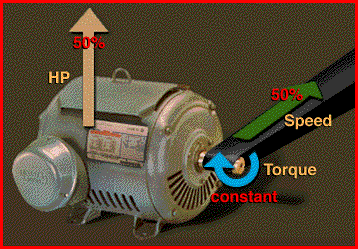For example, if the speed increases by 50%, then the power required to drive the operation will increase 50% while the torque remains constant.

A conveyor operation runs 20 hours a day under differing capacities during the day. Since the conveyor is a constant torque load, the percent loading of the motor is directly proportional to the percent loading of the conveyor belt. Will it help reduce energy costs and improve process control if we install a variable frequency drive?

Specifications
An existing 3 phase, fully loaded 100 horsepower motor with 92% efficiency drives a conveyor belt that has a variable load. The conveyor belt operates 20hours per day. Loading requirements for the conveyor belt vary during the day.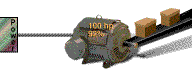Operating Data
• From 6 a.m. to noon, the operation runs at 10% of maximum capacity.
• From noon to 12 midnight, the operation runs at 50% of maximum capacity.
• From 12 midnight to 2 a.m. the operation runs at 100% of maximum capacity.
The motor was sized to drive the conveyor at the maximum power requirement needed for full load capacity of the conveyor. Operation at less than full load requires less power. It also means the efficiency of the motor drops with a decrease in loading.

Energy Costs
The energy costs are four cents per kilowatt-hour with demand charges of five dollars per kW per month for measured usage between 8 a.m. and 10 p.m.

In order to determine the economic feasibility of adding a VFD to this operation, we first compute the energy costs of the current operation.

This is done in three steps. First, we evaluate the loading of the motor over the course of each working day; next, compute the annual energy used, in kilowatt hours; and lastly,calculate the annual energy costs.

To evaluate the loading, we complete the table, taking into account each level of horsepower and efficiency for each load requirement.
To calculate the required output power from the motor to the conveyor at each of the three loading levels, multiply the rated horsepower by the load percentage. Since the conveyor is a constant torque load, the percent loading of the motor is directly proportional to the percent loading of the conveyor belt.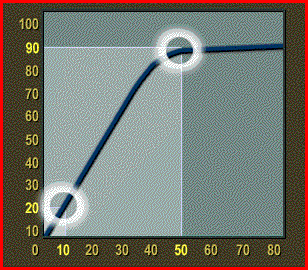Therefore, at 100% load the motor produces 100 horsepower; at 50% load 50 horsepower, and at 10 percent load 10 horsepower.

Because the loading is less than 100% for two of the shifts, the efficiency of the motor during those periods is also less than its full rating of 92%. For the 50% load, the efficiency only drops to 90%; but for the 10% load, the efficiency plummets to 20%. These values are obtained from the manufacturer's load versus efficiency graph.

Next we compute the amount of energy used.

kW Used
In order to compute the annual energy used, we work the formula as shown for each shift. Recall the plant operates 365 days per year.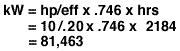For the 6 a.m. to noon shift, the kilowatts used equals 10 horsepower divided by efficiency of .20 times .746, to convert horsepower to kilowatts. Multiply this by the annual operating hours of 2,184 and we get 81,463.

Solving for the other two shifts similarly yields 180,835 kWh and 59,041 kWh.
The total annual Energy Use is 321,339 kilowatt hours.

Annual Energy Cost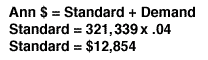The annual energy costs are based on the standard charges plus the demand charges. The standard charges for this operation are computed by taking 321,339 kilowatts per year times the rate of four cents per kilowatt hour. This yields\$12,854.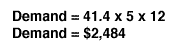The demand period is chargeable and is computed by multiplying the energy use for that period, 41.4 kilowatts times \$5 times 12 months. The charges are \$2,484.

The annual energy costs for this operation is \$15,338.

Next we'll calculate the energy costs for this operation using a VFD.

Savings by Adding variable frequency drive
The advantage of adding a variable frequency drive is that changes in the load have no effect on motor efficiency. We use the motor nameplate efficiency value when calculating energy use at any load.

We use the same formulas to compute energy use. The total energy use is 253,635 kWh, compared to 321,339 before.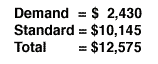The annual cost after adding the variable frequency drive is calculated by adding the demand charges and the standard energy charges. Demand charges are \$2,430 and standard energy charges are \$10,145 for an annual total of \$12,575.

The annual energy costs without the variable frequency drive was \$15,338. The addition of an variable frequency drive yields an Annual Cost Savings of \$2,763.

It is now possible to compute simple payback and look at the lifetime costs.

Simple Payback
To compute simple payback, divide the cost of the VFD, \$25,000, by the cost savings, \$2,763. The payback is approximately 9 years.

Installation of the variable frequency drive makes sense as long as it will last at least through the payback time, 9 years. This variable frequency drive was rated with a service life of 50,000 hours. The conveyor runs a total of 7,280 hours per year.

Dividing the service life of the VFD by the total hours per year gives us 6.9 years life.

The payback is 9 years. The variable frequency drive is expected to last just under 7 years. Installation of the variable frequency drive does not appear to be justified in this application.

Plz Calculate (7 * 5) =
(Your comment will show after approved.)

You may also like:

Category
Featured Articles
What is VFD, How it works? - VFD ...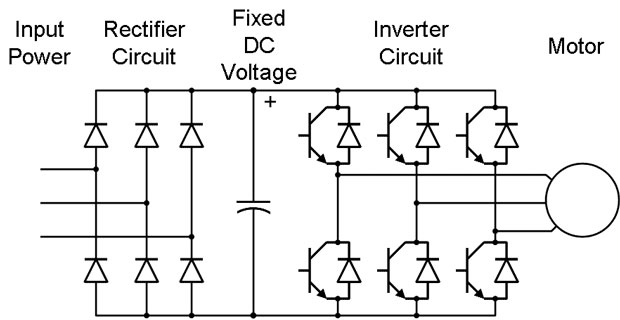VFD is shorted for Variable Frequency Drive (also known as AC Drives and Inverters) -- that's used to make an AC motor working in ...
VFD: Insulated Gate Bipolar Transistor ...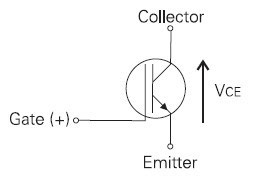IGBT (insulated gate bipolar transistor) provides a high switching speed necessary for PWM VFD operation. IGBTs are capable of ...
Three phase inverters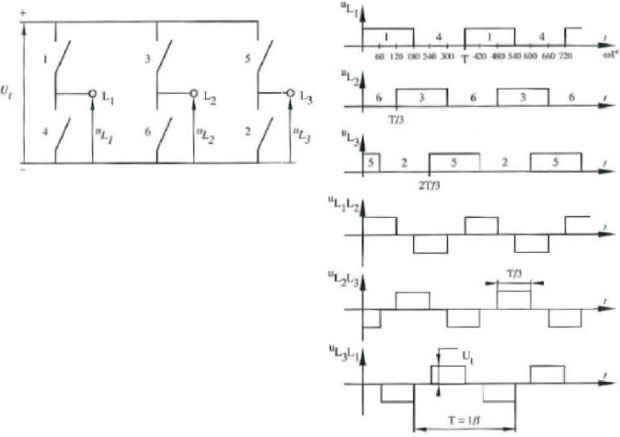In the variable frequency drive rectifier paper, it explains how to go from three phase alternating current voltage to a direct ...
Variable Frequency Drive Harmonics and ... A discussion of the benefits of variable frequency drives often leads to a question regarding electrical harmonic distortion ...
Variable frequency drive Energy saving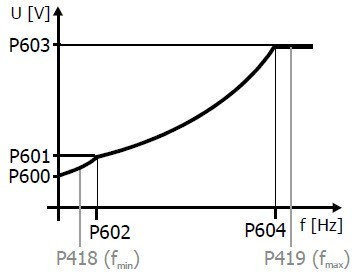Energy can be saved in a VFD by reducing the losses in the electric motor or by reducing the energy consumption of the variable ...VFD manufacturers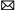buy@vfds.orgtech@vfds.org